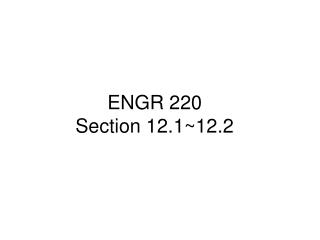DownloadDownload PresentationENGR 220 Section 12.1~12.2

# ENGR 220 Section 12.1~12.2

Download Presentation## ENGR 220 Section 12.1~12.2

- - - - - - - - - - - - - - - - - - - - - - - - - - - E N D - - - - - - - - - - - - - - - - - - - - - - - - - - -
##### Presentation Transcript

1. ENGR 220Section 12.1~12.2

2. Deflection of Beams and Shafts

3. Sign Convention

4. Coordinates v, x and y

5. Curvature related to v and x Non-Linear Second Order Differential Equation. ELASTICA Assumption slope is very small : Engineering Constraints Neglect (dv/dx)^2 term.

6. Deflection Equations

7. Boundary Conditions

8. Example 12.1

9. Determine equations of elastic curve. Specify slope at B and deflection at C 12.26

10. Multiple Loadings : 4 Moment equations 8 constants of Integration 2 Boundary Conditions 6 Continuity Conditions Advanced Methods : * Discontinuity Functions and Singularity Functions * Moment Area Method

11. The fence board weaves between three posts. If the posts remain in the same line, determine maximum bending stress in the board. • The board has a width of 6 in and thickness of 0.5 in. E = 1.6 x 103 ksi. • Assume displacement of each end relative to center of the board is 3 in. 12.13

12. Method of Superposition A steel bar is supported by two springs at its ends A and B. Each spring has a stiffness of 15 kip/ft and is originally unstretched. If the bar is loaded with a force of 3 kip at point C, determine the vertical displacement of the force. Neglect the weight of the bar. Est = 29 (103) ksi. I = 12 in4

13. A picture is taken of a man performing a pole vault. The minimum radius of curvature of the pole is estimated by measurement to be 4.5 meters. If the pole diameter is 40 mm, and is made of glass reinforced plastic for which E = 131 GPa, determine the maximum bending stress in the pole.